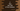# C program to find the LCM of two numbers## How to find the LCM of two numbers in C:

In this post, we will learn how to find the LCM of two numbers in C. LCM or Lowest common multiple is the smallest positive number that is perfectly divisible by both of these numbers. Perfectly divisible means that the remainder is 0 for that division.

This program will take two numbers as inputs from the user and print the LCM.

We will learn different ways to find the LCM.

## Method 1: C program to find the LCM of two numbers using a while loop:

We can find the LCM by using a while loop. Basically, we will take two numbers as inputs from the user and starting from the bigger number, we will start one while loop.

This loop will find the LCM of these two numbers.

• Run the while loop until the value of LCM is found.
• On each iteration, check if the current value is perfectly divisible by both numbers or not. If yes, return that value, else move to next iteration.
• At the end of each iteration, add the larger value to the current value.

Below is the complete program:

``````#include <stdio.h>

int findLCM(int first, int second)
{
int maxValue = first > second ? first : second;
int currentValue = maxValue;

while (1)
{
if (currentValue % first == 0 && currentValue % second == 0)
{
break;
}
currentValue += maxValue;
}
return currentValue;
}

int main()
{
int firstNumber, secondNumber;

printf("Enter the numbers: ");
scanf("%d %d", &firstNumber, &secondNumber);

printf("LCM : %d\n", findLCM(firstNumber, secondNumber));
}``````

Here,

• findLCM method is used to find the LCM. It takes two numbers as its parameters and returns the LCM.
• maxValue is the larger value among the numbers provided in the arguments. This value is assigned as the currentValue.
• The while loop will run for indefinite amount of time. It checks if the currentValue is perfectly divisible by both of these numbers or not. If yes, it breaks from the while loop and returns the currentValue, which is LCM.
• Else, it adds maxValue to currentValue.

If you run this program, it will print output as like below:

``````Enter the numbers: 3 10
LCM : 30``````

## Method 2: C program to find the LCM of two numbers by using GCD in a recursive method:

In this method, we will find the LCM of two numbers by using GCD. GCD stands for greatest common divisor. GCD is equal to the largest number that can divide both numbers perfectly. If we divide the product of two numbers by their GCD, it will return the LCM.

``LCM = (firstNumber * secondNumber)/GCD``

So, we will write one method that will find the GCD. This method will be used by the find LCM method and it will return the LCM.

Below is the complete program:

``````#include <stdio.h>

int findGCD(int first, int second)
{
if (first == 0)
return second;
if (second == 0)
return first;

if (first == second)
return first;

if (first > second)
return findGCD(first - second, second);
return findGCD(first, second - first);
}

int findLCM(int first, int second)
{
int gcd = findGCD(first, second);
return (first * second) / gcd;
}

int main()
{
int firstNumber, secondNumber;

printf("Enter ther numbers: ");
scanf("%d %d", &firstNumber, &secondNumber);

printf("LCM : %d\n", findLCM(firstNumber, secondNumber));
}``````

Here, findGCD is used to find the GCD of two numbers. It takes two numbers as parameters and returns the GCD. findLCM is used to find the LCM of two numbers. It finds the GCD and then divide the product of the numbers by the GCD value to get the LCM.

If you run this program, it will print similar output.